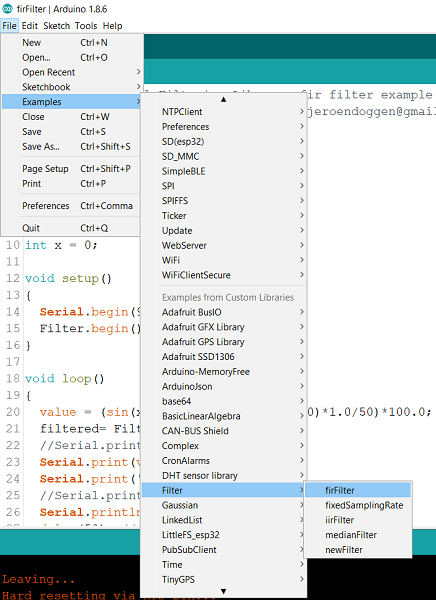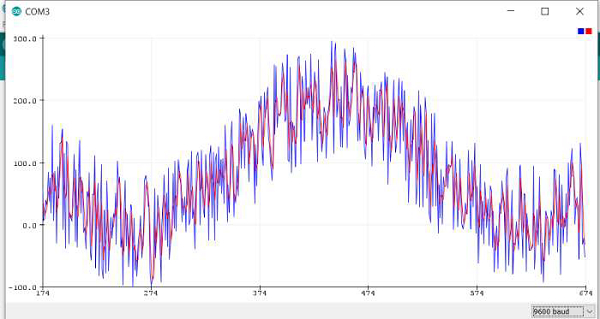# Serial Filtering Library in Arduino

The Serial filtering library in Arduino helps you to apply some low pass filters and the median filter on any incoming data, to give you the filtered output. The GitHub repo of this library can be found here, and it is pretty detailed.

In order to install the library, download the source code from GitHub, and place the 'Filter' folder in the libraries folder of Arduino (on Windows, the path is typically: C:/Users/<YourUserName>/Documents/Arduino/libraries)

Once that is done, in the Arduino IDE, open File→Examples→Filter and pick an example of your choice (firFilter for example)As you can see, the code is quite straightforward.

#include <firFilter.h>

firFilter Filter;

int value;
int filtered;

void setup()
{
Serial.begin(115200);
Filter.begin();
}

void loop()
{
filtered= Filter.run(value);
Serial.print("In: ");
Serial.print(value);
Serial.print(" - Out: ");
Serial.println(filtered);
}

It is taking an incoming analogRead value, applying the filter on it, and returning the output value.

In fact, if you don't have an analog source available, we can test the filter using the random() function. Have a look at the modified example below −

#include <firFilter.h>

firFilter Filter;

int value;
int filtered;
int x = 0;

void setup()
{
Serial.begin(9600);
Filter.begin();
}

void loop()
{
value = (sin(x*3.14/180) + random(100)*1.0/50)*100.0;
filtered= Filter.run(value);
//Serial.print("In: ");
Serial.print(value);
Serial.print(',');
//Serial.print(" - Out: ");
Serial.println(filtered);
}